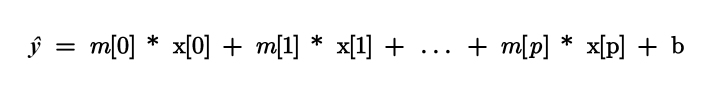# Machine Learning: observe how a linear model works by predicting pricesStudying Machine Learning with a Practical Example. In this article, I’d like to speak about linear models by introducing you to a real project that I made. The project that you can find in my Github consists of predicting the prices of fiat 500.

In this article, I’d like to speak about linear models by introducing you to a real project that I made. The project that you can find in my Github consists of predicting the prices of fiat 500.

The dataset for my model presents 8 columns as you can see below and 1538 rows.

• model: pop, lounge, sport
• engine_power: Kw of the engine
• age_in_days: age of the car in days
• km: kilometres of the car
• previous_owners: number of previous owners
• lat: latitude of the seller (the price of cars in Italy varies from North to South of the country)
• lon: longitude of the seller (the price of cars in Italy varies from North to South of the country)
• price: selling price

During this article, we will see in the first part some concepts about the linear regression, the ridge regression and the lasso regression. Then I will show you the fundamental insights that I found about the dataset I considered and last but not least we will see the preparation and the metrics I used to evaluate the performance of my model.

Part I: Linear Regression, Ridge Regression and Lasso Regression

Linear models are a class of models that make a prediction using a linear function of the input features.

For what concerns regression, as we know the general formula looks like as follows:As you already know x to x[p] represents the features of a single data point. Instead, m a b are the parameters of the model that are learned and *ŷ *is the prediction the model makes.

There are many linear models for regression. The difference between these models is about how the model parameters m and b are learned from the training data and how model complexity can be controlled. We will see three models for regression.

• Linear regression (ordinary least squares) **→ it finds the parameters m** and *b *that minimize the mean squared error between predictions and the true regression targets, y, on the training set. The MSE is the sum of the squared differences between the predictions and the true value. Below how to compute it with scikit-learn.
``````from sklearn.linear_model import LinearRegression

X_train, X_test, y_train, y_test=train_test_split(X, y, random_state=0)
lr = LinearRegression()
lr.fit(X_train, y_train)
print(“lr.coef_: {}”.format(lr.coef_))
print(“lr.intercept_: {}”.format(lr.intercept_))``````

## Data Science With Python Training | Python Data Science Course | Intellipaat

🔵 Intellipaat Data Science with Python course: https://intellipaat.com/python-for-data-science-training/In this Data Science With Python Training video, you...

## Applied Data Analysis in Python Machine Learning and Data Science | Scikit-Learn

Applied Data Analysis in Python Machine learning and Data science, we will investigate the use of scikit-learn for machine learning to discover things about whatever data may come across your desk.

## Data Science Projects | Data Science | Machine Learning | Python

Practice your skills in Data Science with Python, by learning and then trying all these hands-on, interactive projects, that I have posted for you.

## Data Science Projects | Data Science | Machine Learning | Python

Practice your skills in Data Science with Python, by learning and then trying all these hands-on, interactive projects, that I have posted for you.

## Data Science Projects | Data Science | Machine Learning | Python

Practice your skills in Data Science with Python, by learning and then trying all these hands-on, interactive projects, that I have posted for you.